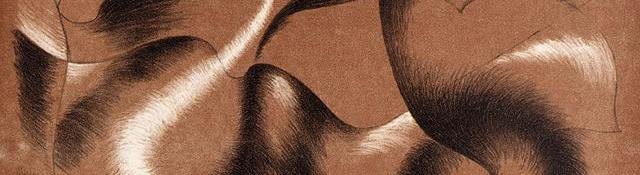## Image Convolutions in OpenCV

Convolutions are a very important tool for anyone interested in signal processing. Image Convolutions is a simpler method to do convolutions on images. And they have a lot of uses too. So of course, OpenCV does have a way to do image convolutions easily and super efficiently!

## OpenCV's Convolution Function

### The C++ convolution function

The one single function that does image convolutions in OpenCV is the Filter2D function. Here's the syntax:

```void filter2D(Mat src,
Mat dst,
int ddepth,
Mat kernel,
Point anchor,
double delta,
int borderType);
```

You'll have to include the cv namespace for the above to work. You can do that with

```using namespace cv;
```

Now for the parameters:

• src: (input) This is the image that you want to convolve.
• dst: (input) This image stores the final result of the convolution. It should be the same size and have the same number of channels as src. This can be the same as src (in place operation is supported).
• ddepth: (input) This is the desired bit depth of the final result (8, 16, 32, etc). It it is negative, the depth is the same as the source image.
• kernel: (input) The convolution kernel used to convolve the source image. This has to be a single channel, floating point matrix. If you want to apply different kernels to different channels, you need to split the channels, and convolve each of them them individually.
• anchor: (input) The relative position of the anchor in the kernel matrix. If this is set to (-1,-1), the center of the kernel is used as the anchor point.
• delta: (input) A value that is added to all pixels after convolution.
• borderType: (input) Possible values for this include:
• BORDER_REPLICATE
• BORDER_CONSTANT
• BORDER_REFLECT_101
• BORDER_WARP
• BORDER_TRANSPARENT
• BORDER_DEFAULT (same as reflect)
• BORDER_ISOLATED

Here's some example code:

```filter2D(img, imgFiltered, -1, kernelLoG, Point(-1,-1), 5.0, BORDER_REPLICATE);
```

The image img is filtered and stored in imgFiltered. The bit depth of imgFiltered will be the same as img (the -1). The convolution will be done using the matrix kernelLog whose anchor is at the center. Also, after the convolution is done, a value of 5.0 will be added to all pixels. The borders are taken care of by replicating pixels around the edges.

### The C image convolution function

The C equivalent of the above function is:

```cvFilter2D(IplImage* src,
IplImage* dst,
CvMat* kernel,
CvPoint anchor);
```

Very similar to the C++ equivalent and a lot simpler too. It doesn't have a lot of extra parameters.

• src: (input) The image you want to convolve.
• dst: (input) The image where the result of the convolution is stored
• kernel: (input) The matrix used to convolve the image.
• anchor: (input) The anchor point of the kernel. If this is (-1,-1) the center of the kernel matrix is used as the anchor.

Pixels "outside" the image are set to the value of the pixel nearest inside the image.

## A few things to keep in mind

The filtering function actually calculates correlation. If you have a symmetrical convolution kernel, the mathematical expressions for correlation and convolution are the same.

If the kernel is not symmetric, you must flip the kernel and set the anchor point to (kernel.cols - anchor.x - 1, kernel.rows - anchor.y - 1). This will calculate the actual convolution.

Also, it isn't always necessary that the filtering will happen on the image directly. If the convolution kernel is large enough, OpenCV will automatically switch to a discrete Fourier transform based algorithm for speedy execution.

## Summary

You got to know how convolutions are done in OpenCV. You learned about the C++ function as well as the C function.

Utkarsh Sinha created AI Shack in 2010 and has since been working on computer vision and related fields. He is currently at Microsoft working on computer vision.